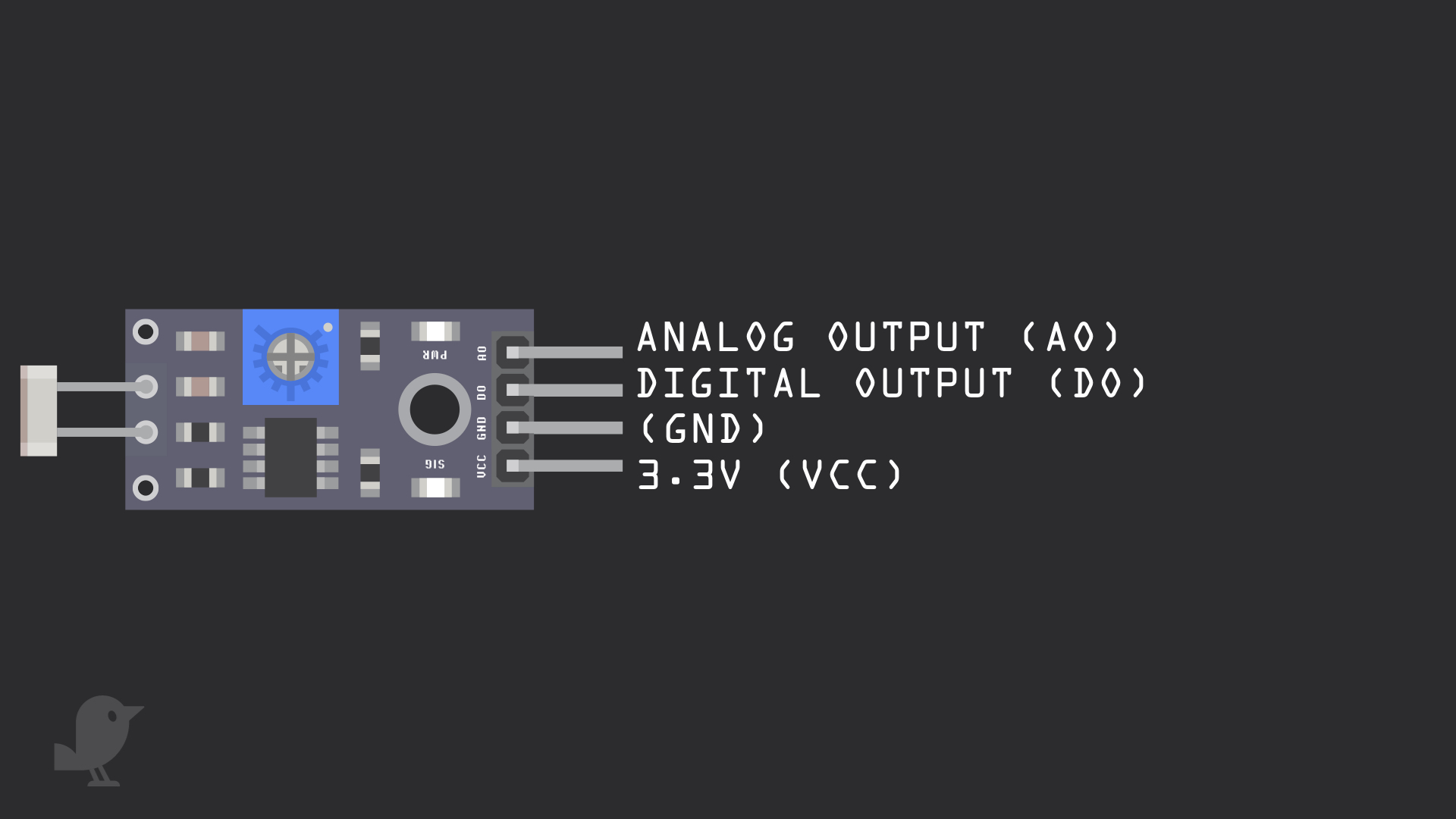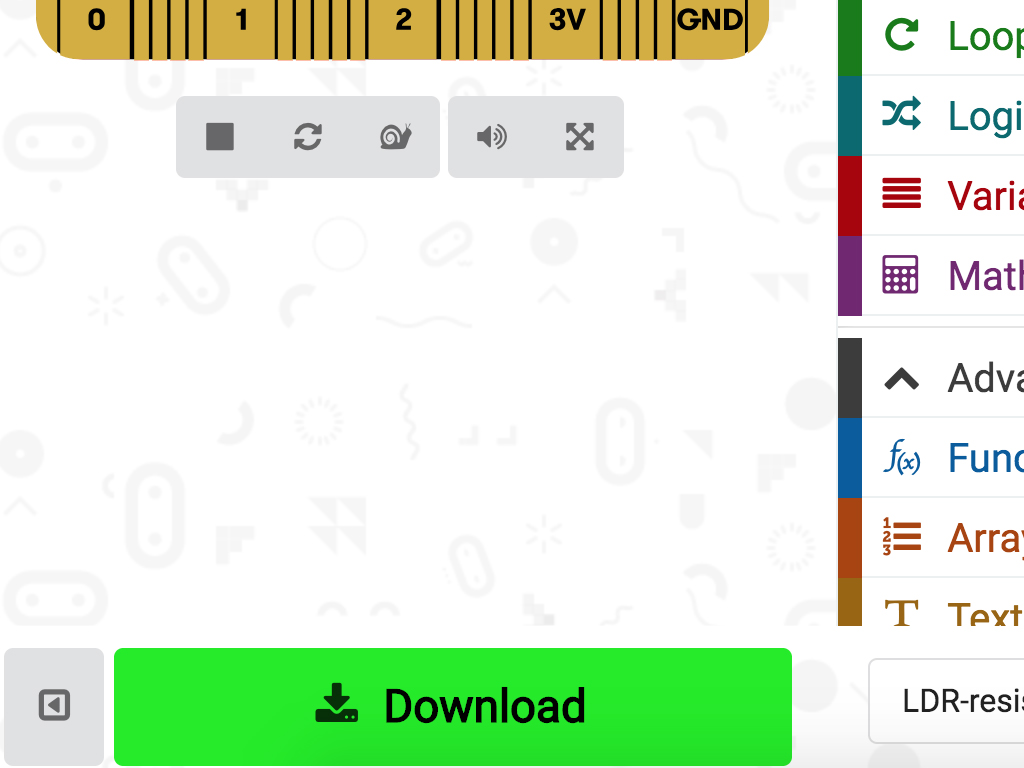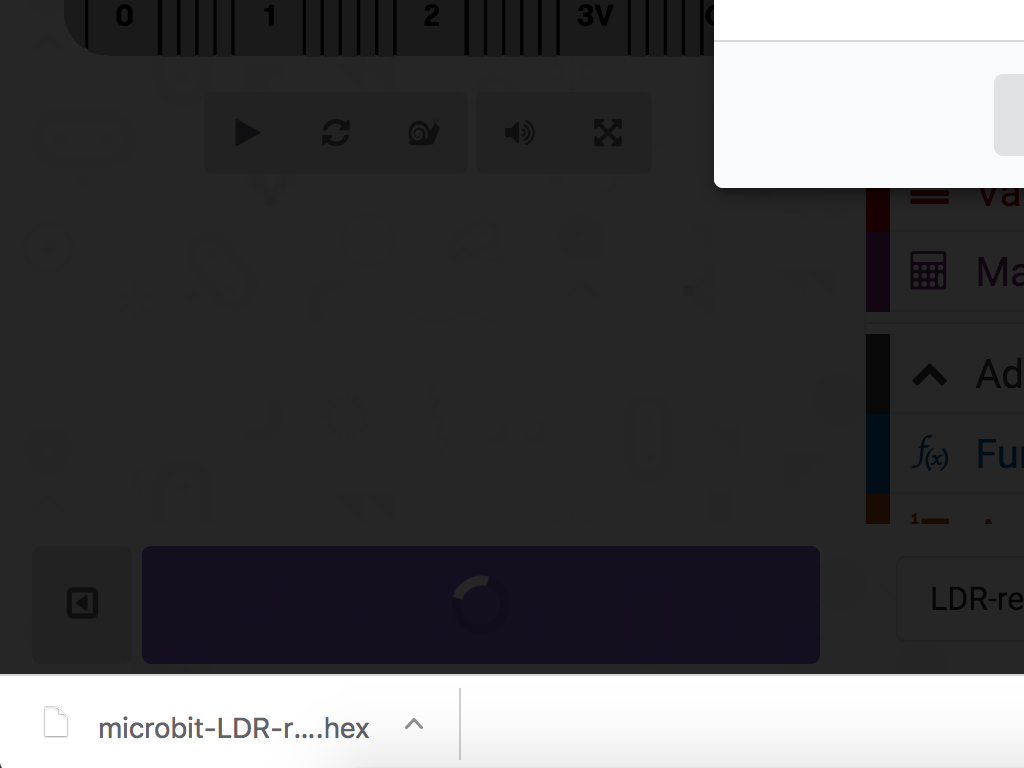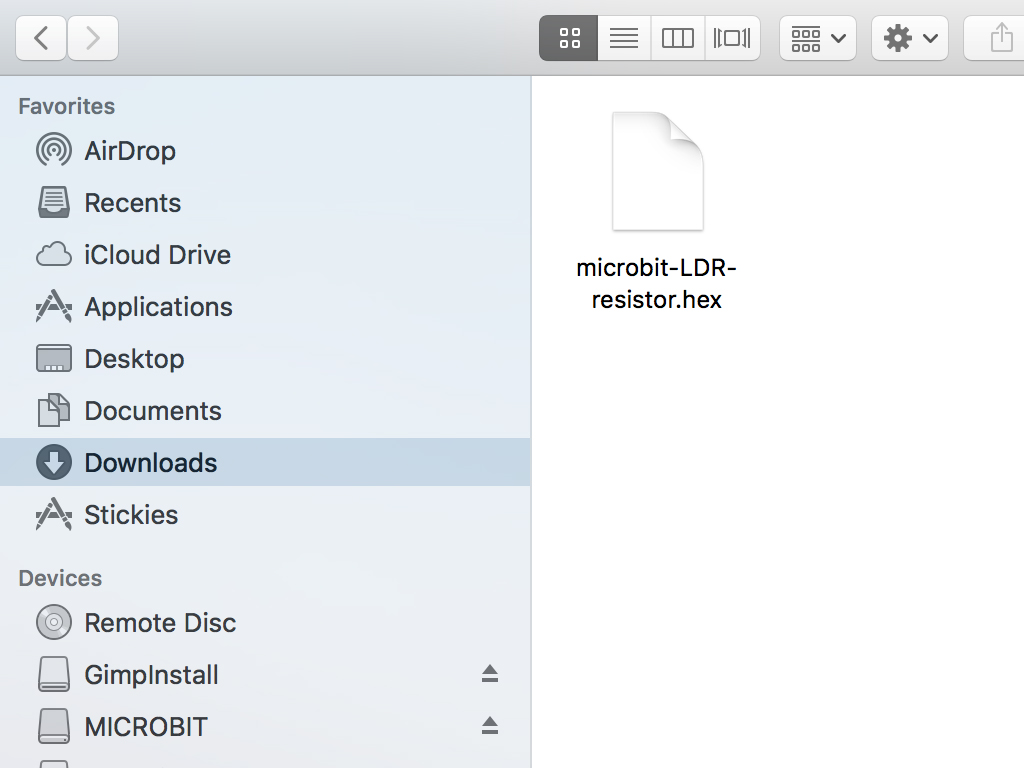## Introduction

Light-dependent resistors are electronic components whose resistance changes with light intensity. They are also called LDRs, photoresistors, or photoconductors.

In this guide, you will learn to connect a light-dependent resistor to the micro:bit, and measure the relative brightness of the environment.

Complete this guide to learn how to use a light-dependent resistor. They are used in all sorts of light-sensing circuits; For instance, they could be used as a sensor in cameras or automatic lights that come on when it gets dark enough.

1. ## Step 1 The Module• Before we begin, let's take a closer look at the light-dependent resistor module. It has four pins:

3.3V  : While 'VCC' stands for Voltage Common Collector, we'll connect the VCC pin to 3.3V on the micro:bit

GND: In electronics, we define a point in a circuit to be a kind of zero volts or 0V reference point, on which to base all other voltage measurements. This point is called  ground or GND.

DO: Digital Output

AO: Analog Output

• Voltage is the difference in potential between two points. As it is difficult to talk about voltage without a reference point, we need another point to compare it to.

7. ## Step 7 Create the variables

``````let threshold = 0
let sensorVal = 0
basic.forever(function () {
threshold = 500
basic.showNumber(sensorVal)
})
``````
• Open up MakeCode editor and start a new project.

• Add the following code to the Javascript interface

8. ## Step 8 The loop

``````let threshold = 0
let sensorVal = 0
basic.forever(function () {
threshold = 400
basic.showNumber(sensorVal)
if (sensorVal > threshold) {
basic.showIcon(IconNames.SmallDiamond)
} else if (sensorVal <= threshold) {
basic.showIcon(IconNames.Target)
}
})
``````
9. ## Step 9 Upload the hex file• Connect your computer to the micro:bit by using a microUSB cable

• Click on the 'Download' button on the bottom left corner of the MakeCode editor